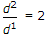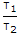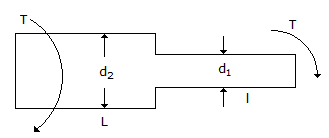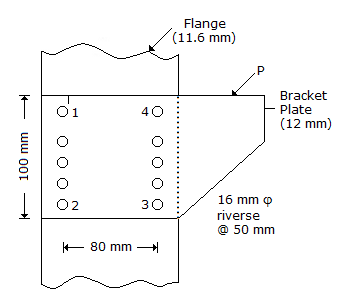# Civil Engineering - UPSC Civil Service Exam Questions

41.

Consider the following statements in respect of a thick cylinder subjected to internal pressure:
1. The stress on an element on the outer wall is unidirectional
2. The stresses on an element on the inner wall are principal stresses.
3. The constants of the Lames equation are positive.
Which of these statements are correct ?

 A. 1 and 2 B. 1 and 3 C. 2 and 3 D. 1, 2 and 3

Explanation:

No answer description available for this question. Let us discuss.

42.

Consider the following statements: An aqueduct is a cross drainage work in which
(a) a canal is carried over the drainage channel
(b) a drainage channel is carried over the canal
(c) both drainage channel and canal are at the same level.
Which of the statements given above is/are correct ?

 A. 1 only B. 1 and 2 only C. 2 and 3 only D. 1, 2 and 3

Explanation:

No answer description available for this question. Let us discuss.

43.

Two shafts of same material and same length are connected in series and subjected to a torque 'T' as shown in the given figure. If, what is the value of the ratio? (Where τ1 and τ2 are the extreme shear stresses in either of two segments)A. 4 B. 2 C. 8 D. 16

Explanation:

No answer description available for this question. Let us discuss.

44.

Which one of the following is the most critical rivet in the joint shown in the figure given?A. No. 1 B. No. 2 C. No. 3 D. No. 4

Explanation:

No answer description available for this question. Let us discuss.

45.

A good brick when immersed in water bath for 24 hours, should not absorb more than

 A. 20% of its dry weight B. 30% of its saturated weight C. 10% of its dry weight D. 20% of its saturated weight